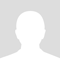# Math Equations, Formulas and Vocabulary

• Area, Volume and Circumference equations:
• Area of a Square
A=S2
• Area of a Triangle
A=1/2bh
• Area of a Rectangle
A=LW
• Right Triangle/Pythagorean Theorem
a2+b2=c2
• Area of Parallelogram
A=bh
• Area of a Trapezoid
A=1/2h(a+b)
• Area of a Circle
A=πr2
• Circumference of a Circle
c=πd or c=2πr
• Volume of a Sphere
V=4/3πr3
• Surface Area of a Sphere
SA=4πr2
• Volume of a Cube
V=s3
• Volume of a Rectangular Solid
V=lwh
• Slope of a line Equations
• Slope-intercept form
y=mx+b
m is the slope
b is the y-intercept
y is a y coordinate on the graph (that coincides with the line)
x is an x coordinate on the graph (that coincides with the line)
• Horizontal line
y=b
• Vertical line
x=a
• Finding slope of line containing points (x1,y1) to (x2,y2)
m=y2-y1
x2-x1
• Distance from one point to another
(x1,y1) to (x2,y2)
d=√(x2-x1)2+(y2-y1)2
• Midpoint of a Line Segment
( x1+x2 y1+y2 )
2           2
x=-b±√b2-4ac
2a
• Math Vocabulary
1. Equilateral Triangle- Three sides of equal length and 3 angles 60° each
2. Scalene Triangle- Three unequal sides and 3 unequal angles
3. Isosceles Triangle- Two sides of equal length and two base angles are equal
4. Commutative Property- a+b=b+a; ab=ba
5. Associative Property- a=(b+c)=(a+b)+c; a(b+c)=ab+ac
6. Distributive Property- a9b+c)=ab+ac
8. Multiplicative Identity- a•1=1•a=a
10. Multiplicative Inverse- a•1/a=1, a≠0\$20p/h

Kayleigh T.

Good with kids with special needs and great with most subjects.